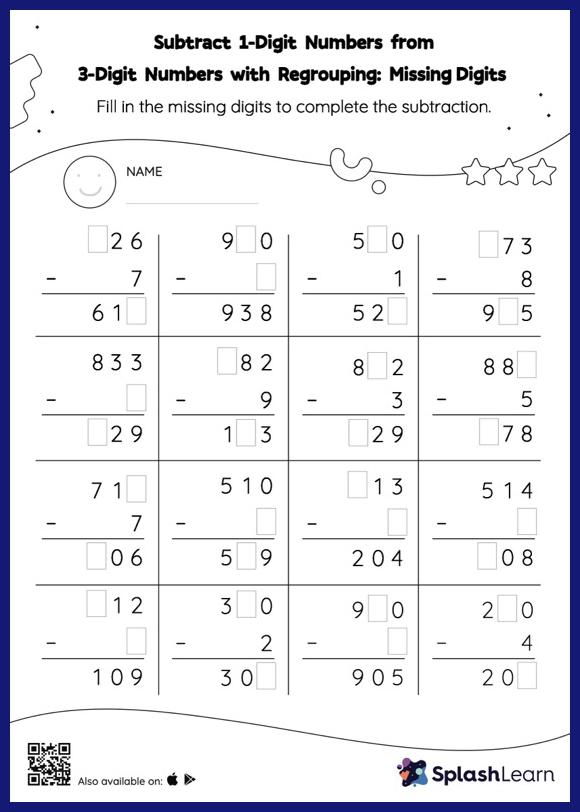# Subtract 1-Digit Numbers from 3-Digit Numbers with Regrouping: Missing Digits Worksheet

Home > Subtract 1-Digit Numbers from 3-Digit Numbers with Regrouping: Missing DigitsTask your little mathematicians to crack the code of subtracting 1-digit numbers from 3-digit numbers with regrouping with this fun worksheet. Students use the count back strategy or the relationship between addition and subtraction to find the missing number in this worksheet. They also need to regroup numbers to get to the answer in subtract 1-digit numbers from 3-digit numbers with regrouping worksheet.